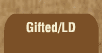Mathematics and Dyslexia

Tips for Learning the Times Tables

Tools for the Times Tables & Workbook

Home > Math > times tables practice > Fours times tables in order

## The fours Times Tables In Order

Numbers multiplied by four are always even. Multiplying by four is the same thing as multiplying by two -- twice. So, if you double something, then double it again, you're multiplying by four.

So, you can figure out that 4 x 3 is twelve by doubling 3 to get 6... then doubling 6 to get 12.

OR... practice this page until you just know them... so when someone says 4 x 3, you think "12."

1. 4 x 0=
2. 4 x 1=
3. 4 x 2=
4. 4 x 3=
5. 4 x 4=
6. 4 x 5=
7. 4 x 6=
8. 4 x 7=
9. 4 x 8=
10. 4 x 9=
11. 4 x 10=
12. 6 x 4=
13. 4 x 7=
14. 8 x 4=
15. 4 x 9=